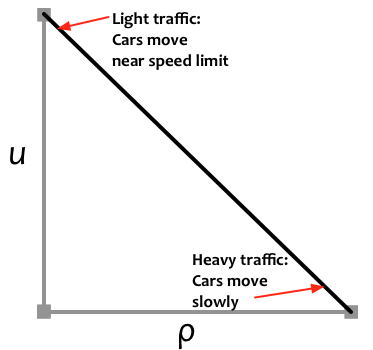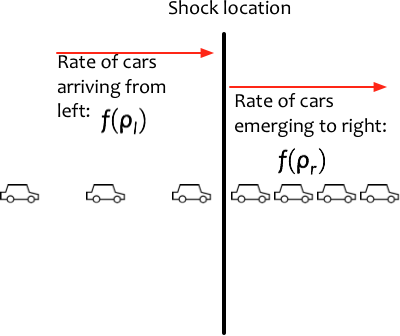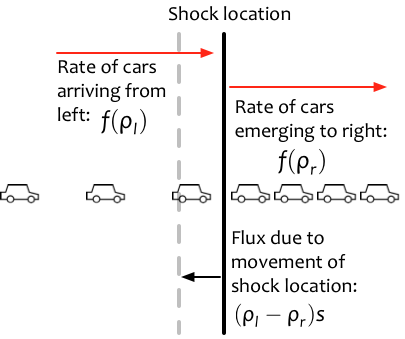# Traffic flow: the Lighthill-Whitham-Richards model¶

In this chapter we investigate a conservation law that models the flow of traffic. This model is sometimes referred to as the Lighthill-Whitham-Richards (or LWR) traffic model (see (Lighthill, 1955) and (Richards, 1956)). This model and the corresponding Riemann problem are discussed in many places; the discussion here is most closely related to that in Chapter 11 of (LeVeque, 2002).

This nonlinear scalar problem is similar to Burgers' equation that we already discussed in Burgers in many ways, since both involve a quadratic (and hence convex) flux function. In this notebook we repeat some of the discussion from Burgers in order to reinforce essential concepts that will be important throughout the remainder of the book.

If you wish to examine the Python code for this chapter, please see:

In :
%matplotlib inline

In :
%config InlineBackend.figure_format = 'svg'
from utils.jsanimate_widgets import interact
from ipywidgets import FloatSlider, fixed
from exact_solvers import traffic_LWR
from exact_solvers import traffic_demos
from IPython.display import Image

Will create JSAnimation figures instead of interactive widget


## The LWR model¶

Recall the continuity equation for any density that is advected with a flow: $$\rho_t + (u\rho)_x = 0.$$
In this chapter, $\rho$ represents the density of cars on a road, traveling with velocity $u$. Note that we're not keeping track of the individual cars, but just of the average number of cars per unit length of road. Thus $\rho=0$ represents an empty stretch of road, and we can choose the units so that $\rho=1$ represents bumper-to-bumper traffic.

We'll also choose units so that the speed limit is $u_\text{max}=1$, and assume that drivers never go faster than this (yeah, right!). If we assume that drivers always travel at a single uniform velocity, we obtain once again the advection equation that we studied in Advection. But we all know that's not accurate in practice -- cars go faster in light traffic and slower when there is congestion. The simplest way to incorporate this effect is to make the velocity a linearly decreasing function of the density: $$u(\rho) = 1 - \rho.$$ Notice that $u$ goes to zero as $\rho$ approaches the maximum density of 1, while $u$ goes to the maximum value of 1 as traffic density goes to zero. Obviously, both $\rho$ and $u$ should always stay in the interval $[0,1]$.

Here is a plot of this velocity function:

In :
Image('figures/LWR-Velocity.png', width=350)

Out:Combining the two equations above, our conservation law says $$\rho_t + (\rho (1-\rho))_x = 0$$ with the flux function $$f(\rho) = \rho(1-\rho)$$ giving the rate of flow of cars. Notice how the flux is zero when there are no cars ($\rho=0$) and also when the road is completely full ($\rho=1$). The maximum flow of traffic actually occurs when the road is half full, as the plot below shows.

In :
f = lambda rho: rho*(1-rho)
traffic_demos.plot_flux(f)


Like the flux in Bugers' equation, the LWR flux is nonlinear, so we again expect to see shock waves and rarefaction waves in the solution. We can superficially make this equation look like the advection equation by using the chain rule to write it in quasilinear form:
$$f(\rho)_x = f'(\rho) \rho_x = (1-2\rho)\rho_x.$$
Then we have
$$\rho_t + (1-2\rho)\rho_x = 0.$$
This is like the advection equation, but with a velocity $1-2\rho$ that depends on the density of cars. The value $f'(\rho)=1-2\rho$ is referred to as the characteristic speed. This characteristic speed is not the speed at which cars move (notice that it can even be negative, whereas cars only drive to the right in our model) Rather, it is the speed at which information is transmitted along the road. Notice that the LWR flux is not convex but concave; because of this, the characteristic speed is a decreasing function of the density.

## Example: Traffic jam¶

What does our model predict when traffic approaches a totally congested ($\rho=1$) area? This might be due to construction, an accident or a red light somewhere to the right; upstream of the obstruction, cars will be bumper-to-bumper, so we set $\rho=1$ for $x>0$ (supposing that traffic has backed up to that point). For $x<0$ we'll assume a lower density $\rho_l<1$. This is another example of a Riemann problem: two constant states separated by a discontinuity. We have
$$\rho(x,t=0) = \begin{cases} \rho_l & x<0 \\ 1 & x>0. \end{cases}$$
What will happen as time goes forward? Intuitively, we expect traffic to continue backing up to the left, so the region with $\rho=1$ will extend further and further to the left. This corresponds to the discontinuity (or shock wave) moving to the left. How quickly will it move? The example below shows the solution (on the left) and individual vehicle trajectories in the $x-t$ plane (on the right).

In :
interact(traffic_demos.jam,
rho_l=FloatSlider(min=0.1,max=0.9,value=0.5,
description=r'$\rho_l$'),
t=FloatSlider(min=0.,max=1.,value=0.5), fig=fixed(0));


Once Loop Reflect

Note that the vehicle trajectory plot above shows the motion of cars moving at velocity $u_l = 1 - \rho_l>0$ as they approach the traffic jam, and at speed $u_r = 1-\rho_r = 0$ to the right of the shock, where the cars are stationary.

Unlike the case of linear advection, the characteristic speeds are different, with $f'(\rho_l) = 1 - 2\rho_l$, which could be either negative or positive depending on $\rho_l$, and $f'(\rho_r) = -1$.

## Speed of a shock wave: the Rankine-Hugoniot condition¶

In the plot above, we see a shock wave (i.e., a discontinuity) that moves to the left as more and more cars pile up behind the traffic jam. How quickly does this discontinuity move to the left?

We can figure it out by putting an imaginary line at the location of the shock, as shown in the next figure.

Let $\rho_l$ be the density of cars just to the left of the line, and let $\rho_r$ be the density of cars just to the right. Imagine for a moment that the line is stationary. Then the rate of cars reaching the line from the left is $f(\rho_l)$ and the rate of cars departing from the line to the right is $f(\rho_r)$. If the line really were stationary, we would need to have $f(\rho_l)-f(\rho_r)=0$ to avoid cars accumulating at the line.

In :
Image('figures/shock_diagram_traffic_a.png', width=350)

Out:However, the shock is not stationary, so the line is moving. Let $s$ be the speed of the shock. Then as the line moves to the left, some cars that were to the left are now to the right of the line. The rate of cars removed from the left is $s \rho_l$ and the rate of cars added on the right is $s \rho_r$, as shown in this figure:

In :
Image('figures/shock_diagram_traffic_b.png', width=350)

Out:So in order to avoid an infinite density of cars at the shock, these two effects need to be balanced:

$$f(\rho_l) - f(\rho_r) = s(\rho_l - \rho_r).$$

This same condition was used for Burgers' equation in Burgers, and is known as the Rankine-Hugoniot condition. It holds for any shock wave in the solution of any hyperbolic PDE (even systems of equations, where the corresponding vector version gives even more information about the structure of allowable shock waves).

Returning to our traffic jam scenario, we set $\rho_r=1$. Then we find that the Rankine-Hugoniot condition gives the shock speed $$s = \frac{f(\rho_l)-f(\rho_r)}{\rho_l-\rho_r} = \frac{f(\rho_l)}{\rho_l-1} = -\rho_l.$$ This makes sense: the traffic jam propagates back along the road, and it does so more quickly if there is a greater density of approaching cars.

## Example: green light¶

What about when a traffic light turns green? At $t=0$, when the light changes, there will be a discontinuity, with traffic backed up behind the light but little or no traffic after the light. With the light at $x=0$, this takes the form of another Riemann problem:
$$\rho(x,t=0) = \begin{cases} 1 & x<0, \\ \rho_r & x>0, \end{cases}$$
with $\rho_r = 0$, for example. In this case we don't expect the discontinuity in density to propagate. Physically, the reason is clear: after the light turns green, the cars in front accelerate and spread out; then the cars behind them accelerate, and so forth. This kind of expansion wave is referred to as a rarefaction wave because the drivers experience a decrease in density (a rarefaction) as they pass through this wave. Initially, the solution is discontinuous, but after time zero it becomes continuous.

### Similarity solutions¶

The exact form of the solution at a green light can be determined by assuming that the solution $\rho(x,t)$ depends only on $x/t$. A solution with this property is referred to as a similarity solution because it remains the same if we rescale both $x$ and $t$ by the same factor. The solution of any Riemann problem is, in fact, a similarity solution. Writing $\rho(x,t) = \tilde{\rho}(x/t)$ we have (with $\xi = x/t$):
\begin{align*} \rho_t & = -\frac{x}{t^2}\tilde{\rho}'(\xi) & f(\rho)_x & = \frac{1}{t}\tilde{\rho}'(\xi) f'(\tilde{\rho}(\xi)). \end{align*}
Thus
\begin{align} \rho_t + f(\rho)_x = -\frac{x}{t^2}\tilde{\rho}'(\xi) + \frac{1}{t}\tilde{\rho}'(\xi) f'(\tilde{\rho}(\xi)) = 0. \end{align}
This can be solved to find \begin{align} f'(\tilde{\rho}(\xi)) & = \frac{x}{t} \end{align}
or, since $f'(\tilde{\rho}) = 1-2\tilde{\rho}$,
\begin{align} \tilde{\rho}(\xi) & = \frac{1}{2}\left(1 - \frac{x}{t}\right). \end{align}
We know that the solution far enough to the left is just $\rho_l=1$, and far enough to the right it is $\rho_r$. The formula above gives the solution in the region between these constant states. For instance, if $\rho_r=0$ (i.e., the road beyond the light is empty at time zero), then
\begin{align} \rho(x,t) & = \begin{cases} 1 & x/t \le -1 \\ \frac{1}{2}\left(1 - x/t\right) & -1 < x/t < 1 \\ 0 & 1 \le x/t. \end{cases} \end{align}

The plot below shows the solution density and vehicle trajectories for a green light at $x=0$.

In :
interact(traffic_demos.green_light,
rho_r=FloatSlider(min=0.,max=0.9,value=0.3,
description=r'$\rho_r$'),
t=FloatSlider(min=0.,max=1.), fig=fixed(0));


Once Loop Reflect